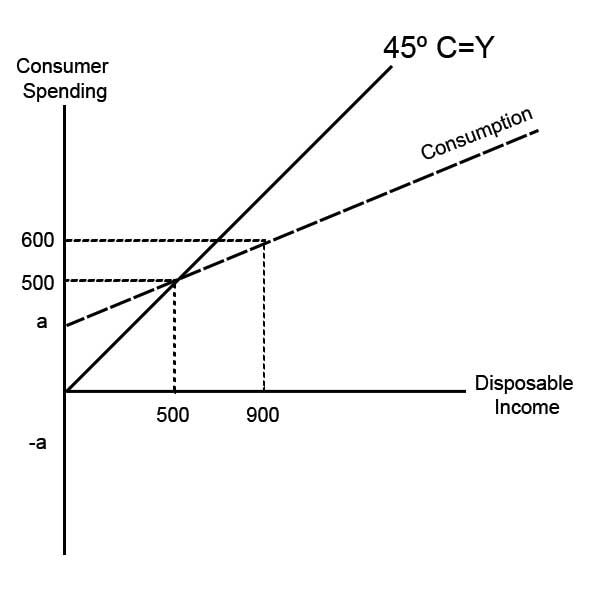top of page### Aggregate Expenditure

#### Marginal Propensity to Consume and Marginal Propensity to Save

The MPC makes up the gradient of the consumption line and the MPS makes up the gradient of the savings line in the consumption function.###### Marginal Propensity to Consume

The Marginal Propensity to Consume (MPC) measures the increase in household consumption from an increase in household income.

To calculate MPC:

MPC = (Change in Consumption)/(Change in Disposable Income)

Can you calculate the MPC from the model below?Change in Consumption (Consumer Spending):

= 600-500

= 100

Change in Disposable Income:

= 900-500

= 400

MPC:

= 100/400

= 0.25

###### Average Propensity to Consume (APC)

Average Propensity to Consume measures the proportion of income spent on consumption.

APC = Total Consumption/Total Disposable Income

Can you find what the APC is from figure 1?

Total Consumption = 600

Total Disposable Income = 900

APC

= 600/900

= 0.67

Note: As income increases, the APC decreases.

###### Marginal Propensity to Save

Marginal Propensity to Save (MPS) measures the increase in savings from a given increase in household income.

MPS = (Change in Savings)/(Change in Disposable Income) OR  MPS = 1 - MPC

Can you find what the MPS is from figure 2?

###### Figure 1###### Figure 2

Method 1 - Find MPC

Change in Consumption

= 625-500

= 125

Change in Disposable Income

= 1000-500

= 500

MPC = 125/500

= 0.25

MPS = 1 -0.25

= 0.75

Method 2 - Find MPS

Change in Savings

= 375 - 0 (Note: the savings level at a disposable income of 500 is 0)

= 375

Change in Disposable Income

= 1000-500

= 500

MPS = 375/500

= 0.75

###### Average Propensity to Save (APS)

Average Propensity to Save (APS) measures the total proportion of income that is saved.

APS = Total Savings/ Total Income

Can you find the APS of figure 2?